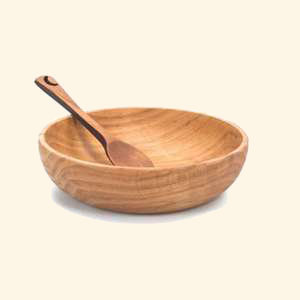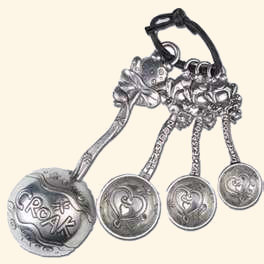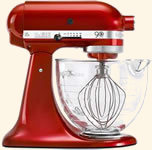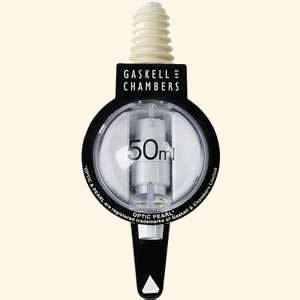Cooking ConversionsPhoto Credit: Barmans

# US Fluid Ounces to Millilitres Conversions (fl oz to ml)

For smaller amounts of liquid in your recipe you will often see fluid ounces (fl oz) or millilitres (ml) as the units. This page will give you the conversions from US fluid ounces to millilites and millilitres to US fluid ounces, if the recipe is from the UK or British Commonwealth you will need the Imperial fluid ounces to millilitres conversion.

For larger liquid measures we also have US pints to litres conversion and US pints to Imperial pints conversion

## US Fluid Ounces to Millilitres Converter

Type a value in either field to convert from US fluid ounces to millilitres (US fl oz to ml) or millilitres to US fluid ounces(ml to US fl oz) (results are accurate to 2 decimal places).

 US Fluid oz Millilitres US fl oz = ml

## Common US Fluid Ounce to Millilitre Conversion Table

Commonly used US fluid ounce to millilitres conversions are given in the table below (results are rounded to the nearest millitre).

 US Fluid Ounces ¼ ½ ¾ 1 1½ 2 3 4 6 8 12 16 Millilitres 7 15 22 30 44 59 89 118 177 237 355 473

## US Fluid Ounces to Millilitres Conversion Formula

The conversion formula for US fluid ounces to millilitres (US fl oz to ml) is 1 US fluid ounce = 29.5735296 millilitres.

## Common Millilitre to US Fluid Ounce Conversion Table

Values commonly used in cooking for millilitre to US fluid ounce conversions are given in the table below (results are rounded to 2 decimal places).

 Millilitres 1 5 10 15 20 25 30 40 50 100 250 500 1000 US Fluid Ounces 0.03 0.17 0.34 0.51 0.68 0.85 1.01 1.35 1.69 3.38 8.45 16.91 33.81

## Millilitre to US Fluid Ounce Conversion Formula

The conversion formula for millilitres to US fluid ounces (ml to US fl oz) is 1 millilitre = 0.03381402 US fluid ounces.

Other liquid measure conversions for cooking